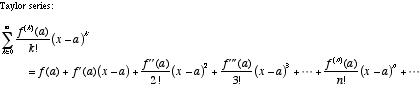index: click on a letter A B C D E F G H I J K L M N O P Q R S T U V W X Y Z A to Z index index: subject areas numbers & symbols sets, logic, proofs geometry algebra trigonometry advanced algebra & pre-calculus calculus advanced topics probability & statistics real world applications multimedia entrieswww.mathwords.com about mathwords website feedback

 Taylor Series The power series in x – a for a function f . Note: If a = 0 the series is called a Maclaurin series.See also